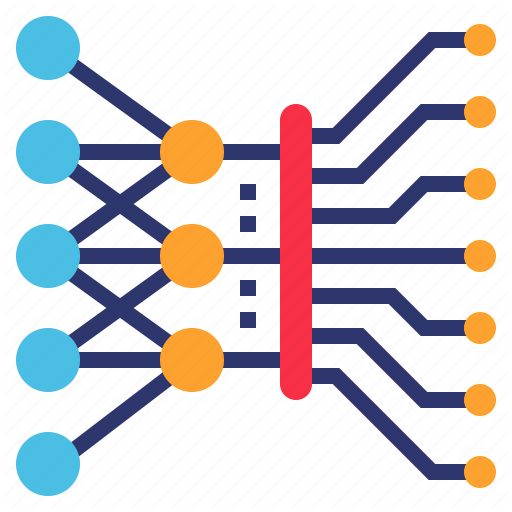# DepthWise Separable Convolution

Original article was published by Parag Chaudhari on Artificial Intelligence on Medium# DepthWise Separable Convolution

Building more efficient neural networks.

Neural networks are beautiful, and the range of tasks they can accomplish is mind-boggling. One of the popular kinds of neural networks is CNN’s convolution neural networks. As they are so wonderful machine learning engineers often have multiple GPUs in the cloud training our models for us. The issue arises when we want to deploy these models on low power devices, such as a mobile phone or an IoT device.

The heart of these models is Convolution.

The way a convolution works is by sliding a filter over our image to generate our features.

This generates the feature map that the succeeding layers can use, instead of working with the raw input. This increases the accuracy of our model as it gets the input in a format it understands better.

Essentially it’s a function that measures overlap between the input image and our chosen kernel

# But what is exactly happening here?

Multiplication. And lots of it. We must first know that multiplication is an expensive operation

What is happening is as the filter slides over the image, we perform multiplications to generate our convoluted filter map

Let’s talk about the cost of this operation.

We can measure this cost by measuring the number of multiplications required.

Here is the cost for one convolution operation.

Let’s calculate the cost of convolution for the entire input.

It will cost us k² x Wo x Ho x M. And that’s for one kernel. For n kernels, it gets as high as k² x Wo x Ho x M x n.

So Cost of convolution= k² x Wo x Ho x M x n

# Let’s take a look at the Depthwise separable convolution.

What makes this different is that it functions in two stages:

1. Depthwise Convolution: The filtering stage.

2. Pointwise Convolution: The combining stage.

Let’s calculate the cost for each of these steps.

# Depthwise Convolution

In depth wise convolution instead of having one filter of depth m, we have m filters of width 1.

In this first stage, we have m kernels of width and height k.

For one convolution we have one_conv = k².

For one channel we have W x H x k².

As we have m channels for all channels, we have DepthConvolutions = W x H x k² x M multiplications.

That’s just for depthwise convolution.

# PointWise Convolution

In pointwise convolution, we have n kernels of shape 1 x 1 x n.

For one operation we have one_conv = 1 x 1 x m where m is the depth of kernel.

So the number of multiplications for one kernel = Wo x Ho x M.

as we have a total of n kernels we end up with

pointwise multiplications=Wo x Ho x M x N

# Now Finally…………

total_multiplication = Depthwise multiplications + Pointwise multiplications

total_multiplication = W x H x k² x M + Wo x Ho x M x N

Comparing it with standard convolution:

Reducing some terms and we end up with:

To bring into perspective how efficient networks with depthwise convolution are let’s consider an example input with n = 512 and k = 5.

If you’d take the inverse of that you’d find that for this scenario you’d find that standard convolutions have 23.86 times more multiplications when compared to depthwise separable convolution. This is a lot of computing power.

Conclusion: Give DepthSeperable Convolution a try, it might allow you to go deeper and wider allowing you to beat the state of the art.

References:

2:https://medium.com/@zurister/depth-wise-convolution-and-depth-wise-separable-convolution-37346565d4ec